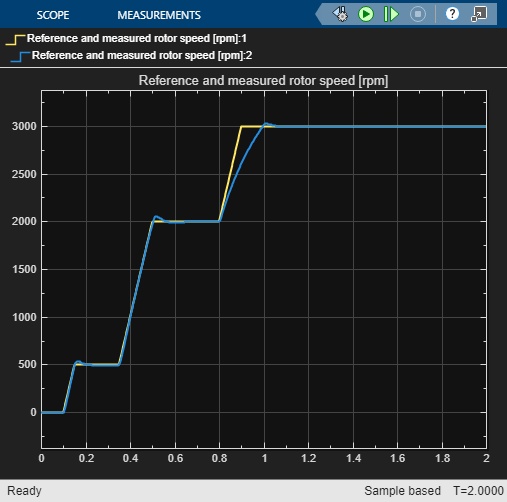# BLDC Motor Speed Control with Cascade PI Controllers

This example shows one of several ways to tune a PID controller for an existing plant in Simulink. Here, you use Closed-Loop PID Autotuner blocks to tune two PI controllers in a cascade configuration. The Autotuner blocks perturb the plant and perform PID tuning based on the plant frequency response estimated near the desired bandwidth. In contrast to the Open-Loop PID Autotuner block, here the feedback loop remains closed and the initial controller gains do not change during the autotuning process.

### BLDC Motor Model

The model in this example uses a 3-phase BLDC motor coupled with a buck converter and a 3-phase inverter power link. The buck converter is modeled with MOSFETs and the inverter with IGBTs rather than ideal switches so that the device on-resistances and characteristics are represented properly. Both the voltages of the DC-DC converter link and the inverter can be controlled by changing the semiconductor gate triggers, which control the speed of the motor.

```mdl = 'scdbldcspeedcontrol'; open_system(mdl) ```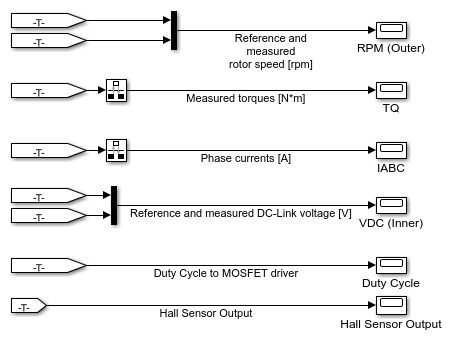The motor model parameters are as follows.

```p = 4; % Number of pole pairs Rs = 0.1; % Stator resistance per phase [Ohm] Ls = 1e-4; % Stator self-inductance per phase, Ls [H] Ms = 1e-5; % Stator mutual inductance, Ms [H] psim = 0.0175; % Maximum permanent magnet flux linkage [Wb] Jm = 0.0005; % Rotor inertia [Kg*m^2] Ts = 5e-6; % Fundamental sample time [s] Tsc = 1e-4; % Sample time for inner control loop [s] Vdc = 48; % Maximum DC link voltage [V] ```

The model is preconfigured to have stable closed-loop operation with two cascaded PI controllers, one for the inner DC link voltage loop, and one for the outer motor-speed loop.

```Kpw = 0.1; % Proportional gain for speed controller Kiw = 15; % Integrator gain for speed controller Kpv = 0.1; % Proportional gain for voltage controller Kiv = 0.5; % Integrator gain for voltage controller ```

The signal for testing the tracking performance is a series of speed ramps from 0-500 RPM, 500-2000 RPM, and 2000-3000 RPM. Simulating the model with initial controller gains shows slow tracking response, indicating that controller recalibration is needed.

```open_system([mdl '/Visualization/RPM (Outer)']) sim(mdl) ```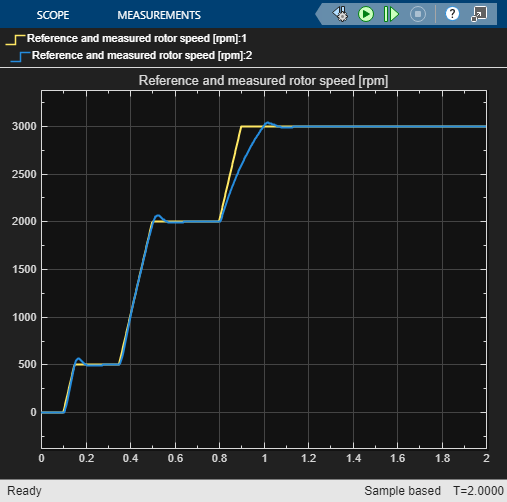### Configure Closed-Loop PID Autotuner Blocks

In this example, you improve the controller performance using Closed-Loop PID Autotuner blocks. These blocks estimate the plant frequency response with the loop closed during the experiment and then tune the controller gains. Examine the Control subsystem to see the Closed-Loop PID Autotuner blocks in the Autotuning Speed and Autotuning Voltage subsystems.

```open_system([mdl '/Control']) ```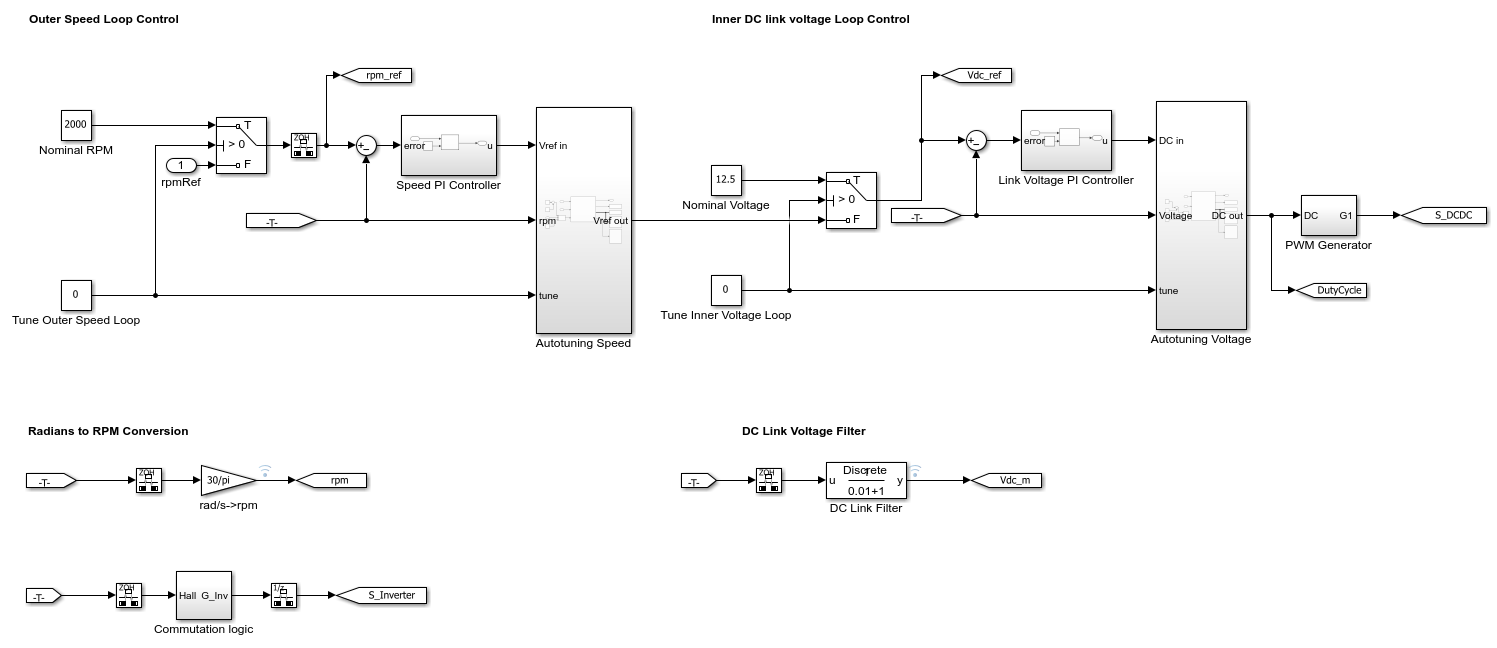Following the typical cascade loop tuning practice, first tune the inner voltage loop with the outer speed loop open. Then, tune the outer speed loop with the inner voltage loop closed.

To specify tuning requirements for the PID controllers, use the parameters on the Tuning tab of each of the PID autotuner blocks. In this example, the controllers are parallel, discrete-time, PI controllers. The controller sample time is 100 microseconds.

A Target Phase Margin of 60 degrees for both controllers gives a good balance between performance and robustness.

For the outer-loop controller, choose a Target Bandwidth of 100 rad/sec. For the inner-loop controller, choose an estimated target bandwidth of 400 rad/sec. These values ensure that the inner-loop controller has a faster response than the outer-loop controller.

The Closed-Loop PID Autotuner block performs a closed-loop experiment to obtain the plant frequency response. You specify parameters for this experiment on the Experiment tab of the block parameters. Here, Plant Sign is Positive, as a positive change in the plant input at the nominal operating point results in a positive change in the plant output, when the plant reaches a new steady state. When the plant is stable, as in this example, the plant sign is equivalent to the sign of its DC gain.

For the amplitude of the sine waves injected during the autotuning process, use 1 to ensure that the plant is suitably excited while remaining within the plant saturation limit. If the amplitude you choose is too small, the autotuner block has difficulty distinguishing the response signals from ripple in the power electronics circuits.

### Tune Inner-Loop PI Controller

For tuning cascade controllers, set up the model for tuning the inner voltage loop first, followed by the outer speed loop.

To enable the tuning process for the inner-loop controller, in the Autotuning Voltage subsystem, set the Tune Inner Voltage Loop constant block value to 1. Setting this value opens the outer loop and configures the inner loop to uses a constant nominal voltage reference of 12.5 instead.

```set_param([mdl '/Control/Tune Inner Voltage Loop'],'Value','1') ```

Also, to disable outer loop tuning, set the Tune Outer Speed Loop constant block value to 0.

```set_param([mdl '/Control/Tune Outer Speed Loop'],'Value','0') ```

This setting enables the Closed-Loop PID Autotuner block which is configured to run a closed-loop tuning experiment from 1 to 1.8 seconds of simulation time. The plant uses the first second to reach a steady-state operating condition. A good estimate for a closed-loop experiment duration is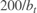, where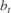is the target bandwidth. You can use the `% conv` output of the Closed-Loop PID Autotuner block to monitor the progress of the experiment and stop it when the `% conv` signal stabilizes near 100%.

Run the simulation. When the experiment concludes, the Closed-Loop PID Autotuner block returns the tuned PID controller gains for the inner voltage loop. The model sends them to the MATLAB workspace as the array `VoltageLoopGains`.

```close_system([mdl '/Visualization/RPM (Outer)']) open_system([mdl '/Visualization/VDC (Inner)']) sim(mdl) ```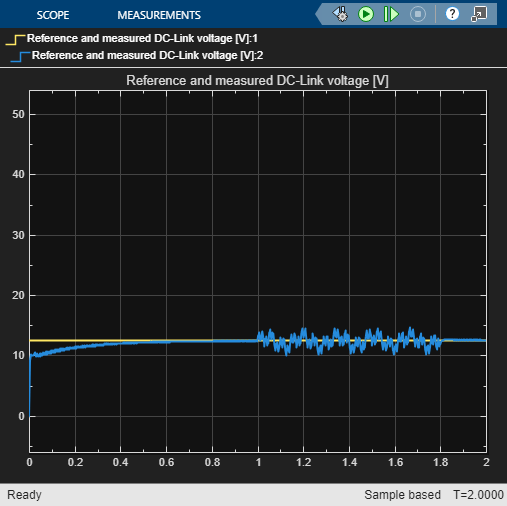Update the inner loop PI controller with the new gains.

```Kpv = VoltageLoopGains(1); Kiv = VoltageLoopGains(2); ```

### Tuning Outer Loop PI Controller

Next, tune the outer speed loop. In the Autotuning Voltage subsystem, change the value of the Tune Inner Voltage Loop constant block value to 0, which disables the inner voltage loop tuning. The inner-loop controller uses the newly tuned gains, `Kpv` and `Kiv`.

```set_param([mdl '/Control/Tune Inner Voltage Loop'],'Value','0') ```

Similarly, in the Autotuning Speed subsystem, change the Tune Outer Speed Loop constant block value to 1, which enables the outer speed loop tuning. For this loop, use a closed-loop autotuning duration of 0.9 seconds, beginning at 1 second. The nominal speed for tuning is 2000 RPM.

```set_param([mdl '/Control/Tune Outer Speed Loop'],'Value','1') ```

Run the simulation again. When the experiment concludes, the Closed-Loop PID Autotuner block returns the tuned PID controller gains for the outer speed loop. The model sends them to the MATLAB workspace as the array `SpeedLoopGains`.

```close_system([mdl '/Visualization/VDC (Inner)']) open_system([mdl '/Visualization/RPM (Outer)']) sim(mdl) ```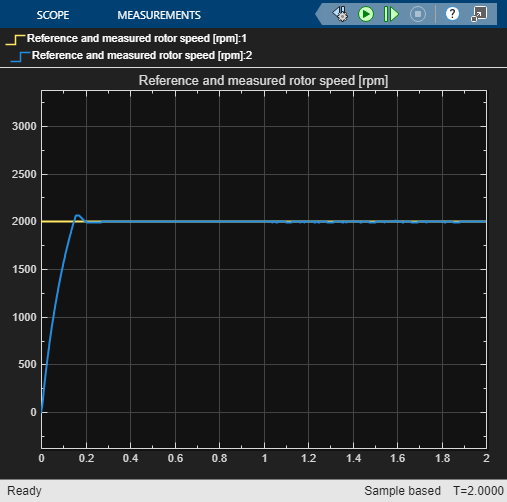Update the outer-loop PI controller with the new gains.

```Kpw = SpeedLoopGains(1); Kiw = SpeedLoopGains(2); ```

### Improved Tracking Performance After Autotuning

To check the tuned controller performance, disable tuning in both loops.

```set_param([mdl '/Control/Tune Inner Voltage Loop'],'Value','0') set_param([mdl '/Control/Tune Outer Speed Loop'],'Value','0') ```

The tuned gains result in better tracking of the test ramp signals.

```sim(mdl) ```